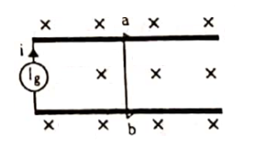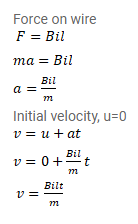# The current generator Ig, shown in figure, sends a constant current

Question:

The current generator $I_{g}$, shown in figure, sends a constant current i through the circuit. The wire ab has a length I and mass $\mathrm{m}$ and can slide on the smooth, horizontal rails connected to $I_{g}$. The entire system lies in a vertical magnetic field $B$. Find the velocity of the wire as a function of time.Solution: